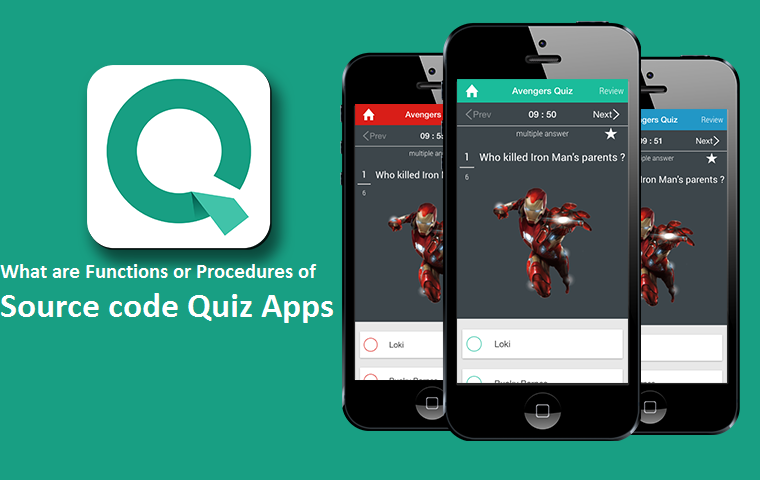# What are Functions or Procedures of Source code Quiz Apps?Written by

Quiz app is created as a project; it is like Mobile App that is implementing in Android platform. This app and guidelines are used to develop code. ERD, SRD and other software needs for quiz app are mentioned in the report file.  Android main and mini-project are also available with Source Code Quiz AppSimple example to explain source code quiz apps

In this blog post, I have to bring some quiz example. We’ve explained source code. This app is in single layout.

1. Create a project

As you’re already familiar with drill system! You should create project with basic configurations. We have created it with some variables or attributes as mentioned below:-

Name of Project – TrivialApp

Name of App- TrivialApp

Name of Package- com.example.TrivialApp

Name of Acitvity- Quiz

1. Now you must define a class to mention questions with list of answers

We’ve defined a class with questions due to important factors, each time we can mention these question, it is also easy to mention an object than set of characters or string.

package com.examplea.triviality;
SETTING UP VARIABLES

public Question()
{
IDa=0;
QUESTIONa=“”;
OPTAa=“”;
OPTBa=“”;
OPTCa=“”;
}
public Question(String qUESTIONa, String oPTAa, String oPTBa, String oPTCa,

QUESTION = qUESTIONa;
OPTA
= oPTAa;
OPTB
= oPTBa;
OPTC
= oPTCa;
}
public int getIDa()
{
return ID;
}
public String getQUESTIONa() {
return QUESTION;
}
public String getOPTAa() {
return OPTA;
}
public String getOPTBa() {
return OPTB;
}
public String getOPTCa() {
return OPTC;
}
}
public void setIDa(int id)
{
ID
=id;
}
public void setQUESTIONa(String qUESTION) {
QUESTION
= qUESTION;
}
public void setOPTAa(String oPTA) {
OPTA
= oPTA;
}
public void setOPTBa(String oPTB) {
OPTB
= oPTB;
}
public void setOPTCa(String oPTC) {
OPTC
= oPTC;
}
}}

3. Now you may recall the database helper as it was introduced through me due to previous list. As DB helper is used as Quiz-helper. It has functions to make a database, tables and insert queries. You need to delete or modify questions when required. The source code is for DB-helper. I have passed it quickly as we have explained in this article.

package com.example.triviality;
import java.util.ArrayList;
import java.util.List;
import android.content.ContentValues;
import android.content.Context;
import android.database.Cursor;
import android.database.sqlite.SQLiteDatabase;
import android.database.sqlite.SQLiteOpenHelper;
public class DbHelper extends SQLiteOpenHelper {
private static final int DATABASE_VERSION = 1;
private static final String DATABASE_NAME = “triviaQuiz”;
private static final String TABLE_QUEST = “quest”;
private static final String KEY_ID = “id”;
private static final String KEY_QUES = “question”;
private static final String KEY_ANSWER = “answer”; private static final String KEY_OPTA= “opta”; //option a
private static final String KEY_OPTB= “optb”; //option b
private static final String KEY_OPTC= “optc”; //option c
private SQLiteDatabase dbase;
public DbHelper(Context context) {
super(context, DATABASE_NAME, null, DATABASE_VERSION);
}
@Override
public void onCreate(SQLiteDatabase db) {
dbase=db;
String sql = “CREATE TABLE IF NOT EXISTS ” + TABLE_QUEST + ” ( ”
+ KEY_ID + ” INTEGER PRIMARY KEY AUTOINCREMENT, ” + KEY_QUES
+ ” TEXT, ” + KEY_ANSWER+ ” TEXT, “+KEY_OPTA +” TEXT, ”
+KEY_OPTB +” TEXT, “+KEY_OPTC+” TEXT)”;
db.execSQL(sql);
//db.close();
}
{
}
@Override
public void onUpgrade(SQLiteDatabase db, int oldV, int newV) {
db.execSQL(“DROP TABLE IF EXISTS ” + TABLE_QUEST);
onCreate(db);
}
define variables }
public List<Question> getAllQuestions() {
List<Question> quesList = new ArrayList<Question>();
String selectQuery = “SELECT * FROM ” + TABLE_QUEST;
Cursor cursor = dbase.rawQuery(selectQuery, null);
if (cursor.moveToFirst()) {
do {
Question quest = new Question();
quest.setID(cursor.getInt(0));

} while (cursor.moveToNext());
}
// return quest list
return quesList;
}
public int rowcount()
{
int row=0;
String selectQuery = “SELECT * FROM ” + TABLE_QUEST;
SQLiteDatabase db = this.getWritableDatabase();
Cursor cursor = db.rawQuery(selectQuery, null);
row=cursor.getCount();
return row;
}
} Now add queries to database